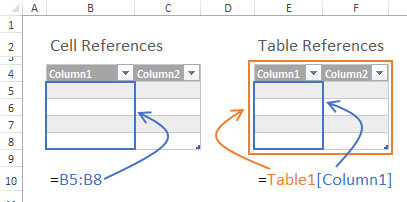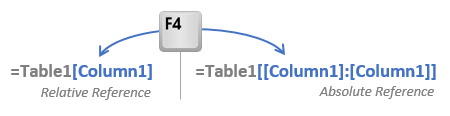# Absolute Structured References in Excel Tables

Absolute Structured References in Excel Tables

Bottom line: This post will explain a trick for creating absolute structured references in Excel Table formulas.  Also known as locking or anchoring the column references.

Skill level: Beginner

## Intro

Structured Reference Tables are great for creating clean, easy to read formulas.  But creating absolute references to the columns (aka anchoring the columns) in the formula is a bit tricky.

## Quick GuideDuplicate the column references as if referring to multiple columns.  Absolute references to:

• One column in the same or other table: table1[[column1]:[column1]]
• One cell in the same row as the formula: table1[@[column1]:[column1]]
• Table names must be used even if the reference and formula cell are in the same table.
• You must drag these formulas across columns to maintain the absolute reference (copy & paste does not work).

I have developed an add-in allows you to use the F4 key on the keyboard to create absolute/relative references.  You can download the Absolute Reference Add-in here.

_

## Overview of Tables

In Excel 2007, Microsoft introduced Structured Reference Tables (aka Tables).  These tables have a lot of great features that make it easier to work with and analyze data sets.  Tables include a new syntax for referring to table columns in formulas.Instead of using cell addresses with column letters and row numbers, Tables allow you to reference cells or ranges with the table and column name.  The major benefit of this is that formulas are much easier to type and read when they refer to descriptive attributes of the table (table and column names).

If you are not familiar with the Tables feature yet, checkout this video:

Excel Tables Tutorial: Beginners Guide for Windows & MacThe video is an in-depth tutorial on how to create and use tables.  I explain 10 awesome features that will save you lots of time when working with your data.

_

### Problem with Absolute References in Tables

However, there is no direct way to create an absolute reference for a table reference in a formula.  By default, all table references are absolute and have the following behavior when dragged or copied:

• Formula dragged across columns: Column references change by referring to the next column to the right.
• Formula copy/pasted across: Column references remain static; do not change when copy/pasted.

When your formula needs to contain a combination of absolute and relative references, there is no way to drag or copy the formula across and keep the references correct.  Dragging the formula across will make all the references change, and copy/pasting will make all the references stay the same.

### Example

I’m going to use the following SUMIF formulas as an example.  You can download the example workbook below to follow along.

• Cell Reference (cell G5): =SUMIF(\$E\$12:\$E\$23,\$E5,G\$12:G\$23)
• Structured Table Reference (cell G6): =SUMIF(t_Data[Color],[@Color],t_Data[Q1 Units])

These formulas reference the exact same cells in the worksheet.  The structured reference formula contains the table and column names instead of the cell references.  This table style was introduced in Excel 2007, and carries through to Excel 2010 and 2013.  In my opinion the formula is much easier to read because you know exactly what you are summing.  In this case, we are summing all rows that contain the Color in the t_Data table that match the color in the same row [@Color] as the formula for [Q1 Units].

The goal is to drag/copy this formula to the right so we can see the results for Q2, Q3, and Q4.  And we don’t want to waste time retyping the formula.

In the cell reference formula we are able to anchor column E in the first argument by adding a \$ sign in front of the E (\$E\$12:\$E\$23).  So when you copy or drag the formula across the columns, the reference will remain anchored to column E.  This is an absolute reference.  If we perform this same copy/drag operation with the structure reference formula, the column references will change for that same argument.  The “t_Data[Color]” reference will change to “t_Data[Region]” which is one column to the right.  This is considered a relative reference and not what we want for this formula.

### The Solution

To create an absolute reference with structured references you need to add an additional and duplicate column reference.

t_Data[[Color]:[Color]]

Duplicating the column reference will anchor the reference when dragging across columns.  Here are the full formulas with relative and absolute references.

• Relative: =SUMIF(t_Data[Color],[@Color],t_Data[Q1 Units])
• Absolute: =SUMIF(t_Data[ [Color]:[Color] ],t_Summary[@ [Color]:[Color] ],t_Data[Q1 Units])

In the absolute formula you’ll notice that I anchored the first two arguments.  The [@Color] column needs be anchored as well.

To anchor a row reference you need to put the @ symbol before the duplicate column reference and wrap it all in brackets.  The table name is also required, even when the reference is in the same table as the formula.  So the row reference looks like:

t_Summary[@[Color]:[Color]]

This is an anchored reference to one cell in the same row as the formula.

The Absolute Reference Add-in helps make this process a lot faster by allowing you to use the F4 key on the keyboard to toggle between absolute/relative references in table formulas.It is very simple and easy to use, but packed with automated features so you can update your formulas in under 3 seconds!Absolute References in Structured Reference Tables.xlsx (14.8 KB)